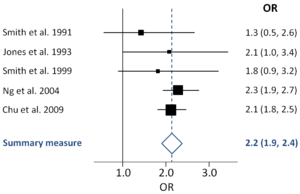# Forest plot

For private plots producing forest products, see Woodlot.An example forest plot of five odds ratios (squares, proportional to weights used in meta-analysis), with the summary measure (centre line of diamond) and associated confidence intervals (lateral tips of diamond), and solid vertical line of no effect. Names of (fictional) studies are shown on the left, odds ratios and confidence intervals on the right.

A forest plot, also known as a blobbogram, is a graphical display of estimated results from a number of scientific studies addressing the same question, along with the overall results. It was developed for use in medical research as a means of graphically representing a meta-analysis of the results of randomized controlled trials. In the last twenty years, similar meta-analytical techniques have been applied in observational studies (e.g. environmental epidemiology) and forest plots are often used in presenting the results of such studies also.

Although forest plots can take several forms, they are commonly presented with two columns. The left-hand column lists the names of the studies (frequently randomized controlled trials or epidemiological studies), commonly in chronological order from the top downwards. The right-hand column is a plot of the measure of effect (e.g. an odds ratio) for each of these studies (often represented by a square) incorporating confidence intervals represented by horizontal lines. The graph may be plotted on a natural logarithmic scale when using odds ratios or other ratio-based effect measures, so that the confidence intervals are symmetrical about the means from each study and to ensure undue emphasis is not given to odds ratios greater than 1 when compared to those less than 1. The area of each square is proportional to the study's weight in the meta-analysis. The overall meta-analysed measure of effect is often represented on the plot as a dashed vertical line. This meta-analysed measure of effect is commonly plotted as a diamond, the lateral points of which indicate confidence intervals for this estimate.

A vertical line representing no effect is also plotted. If the confidence intervals for individual studies overlap with this line, it demonstrates that at the given level of confidence their effect sizes do not differ from no effect for the individual study. The same applies for the meta-analysed measure of effect: if the points of the diamond overlap the line of no effect the overall meta-analysed result cannot be said to differ from no effect at the given level of confidence.

Forest plots date back to at least the 1970s. One plot is shown in a 1985 book about meta-analysis.:252 The first use in print of the expression "forest plot" may be in an abstract for a poster at the Pittsburgh (US) meeting of the Society for Clinical Trials in May 1996. An informative investigation on the origin of the notion "forest plot" was published in 2001. The name refers to the forest of lines produced. In September 1990, Richard Peto joked that the plot was named after a breast cancer researcher called Pat Forrest and as a result the name has sometimes been spelled "forrest plot".

4. Steff Lewis & Mike Clarke (June 2001). "Forest plots: trying to see the wood and the trees". BMJ. 322 (7300): 1479–1480. doi:10.1136/bmj.322.7300.1479. PMC 1120528. PMID 11408310.